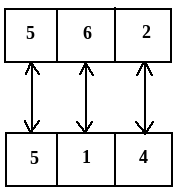# What number is opposite 3 in the figure shown below? The given two positions are of the same dice whose each surface bears a number among 1, 2, 3, 4, 5 and 6.Option 1)   2   Option 2)   4   Option 3)   5   Option 4)   7   Option 5)   6

In the above three diagrams,

To find out the opposite faces we will wright all the elements in the clockwise direction starting with the common element in any two figure.

So taking figure (i) & figure (ii) for the above experiment,Here in this diagram we know that 6 is opposite to 1 & 2 is opposite to 4.

Then numbers left = 3 & 5

So we can say that number opposite to 3 = 5

Exams
Articles
Questions